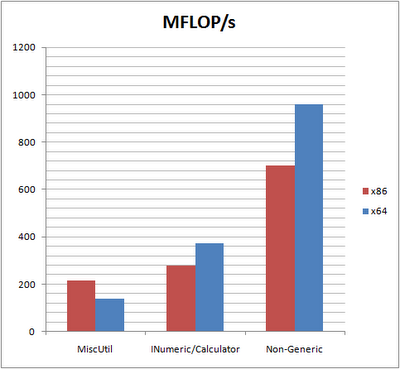## Saturday, April 11, 2009

### Generic .NET Math — First Benchmarks

As promised, I did some first benchmarking of different methods to implement generic arithmetic for .NET. I implemented simple vector classes using the Operator<T> class found in the MiscUtil library, another generic vector class using the approach described in my last posting (using INumeric/Calculators) and compared that to a completely non-generic vector implementation. The benchmarking method solved the following recursive problem for i = 100,000,000 on a Core 2 Quad Q9550:
sum_i := sum_(i-1) + <u_i, v> + <u_i, t>
u_i := u_(i-1) * sum_iAs the figure above shows, the non-generic version is far superior to the other implementations, especially in Long Mode (x64). The x86 version of the interface constraint approach (INumeric/Calculator) is only a tiny bit faster than MiscUtil’s Operator<T> class using runtime code generation. For x64 however, it’s about twice as fast.

References: